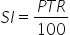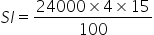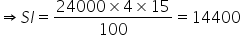Maths-
General
Easy

Question

# Arun borrowed Rs 24,000 from Rahul at the rate of 15% per annum simple interest for 4 years. Find the simple interest and the total amount that has to be paid at the end of 4 years.Hint:

## The correct answer is: 38400 Rupees

### Complete step by step solution:We calculate simple interest by the formula,…(i)where P is Principal amount, T is number of years and R is rate of interestMoney borrowed by Arun from Rahul = P = 24000 RsHere, we have T = 4,R = 15% and P = 24000On substituting the known values in (i), we getWe have SI = 14400 Rupees.We know the formula for total amount = A = P + SI…(ii)where A is the total amount, P is the principal amount and SI is simple interest.On substituting the known values in (ii), we get A = 24000 + 14400 = 38400Hence total amount to be paid after 4 years = A = 38400 Rupees.#### With Turito Foundation.#### Get an Expert Advice From Turito.